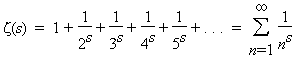A# Riemann zeta function

 We may – paraphrasing the famous sentence of George Orwell – say that "all mathematics is beautiful, yet some is more beautiful than the other." But the most beautiful in all mathematics is the zeta function. There is no doubt about it. —Krzysztof Maslanka, Polish cosmologist

The Riemann zeta function is one of the most profound and mysterious objects in modern mathematics. From it has sprung the Riemann hypothesis and all that this conjecture seems to imply. The Riemann zeta function is closely tied to the distribution of prime numbers. It is an extension of the Euler zeta function, first studied by Leonhard Euler, which is the sumEuler found that this function is linked to the occurrence of prime numbers by the following fundamental relationship:

ζ(s) = 1 + 1/2s + 1/3s + 1/4s + ... = 2n/(2n - 1) × 3n/(3n - 1) × 5n/(5n - 1) × 7n/(7n - 1) × ...

The Riemann zeta function extends the definition of Euler's zeta function to all complex numbers. Zeta functions, in general, take the form of infinite sum of negative powers.